# 8.8 Vectors  (Page 10/22)

 Page 10 / 22

Suppose a body has a force of 3 pounds acting on it to the left, 4 pounds acting on it upward, and 2 pounds acting on it 30° from the horizontal. What single force is needed to produce a state of equilibrium on the body? Draw the vector.

5.1583 pounds, 75.8° from the horizontal

## Non-right Triangles: Law of Sines

For the following exercises, assume $\text{\hspace{0.17em}}\alpha \text{\hspace{0.17em}}$ is opposite side $\text{\hspace{0.17em}}a,\beta \text{\hspace{0.17em}}$ is opposite side $\text{\hspace{0.17em}}b,\text{\hspace{0.17em}}$ and $\text{\hspace{0.17em}}\gamma \text{\hspace{0.17em}}$ is opposite side $\text{\hspace{0.17em}}c.\text{\hspace{0.17em}}$ Solve each triangle, if possible. Round each answer to the nearest tenth.

$\beta =50°,a=105,b=45$

Not possible

$\alpha =43.1°,a=184.2,b=242.8$

Solve the triangle.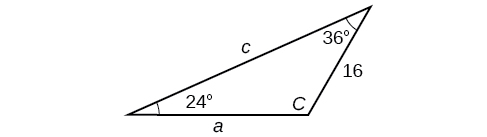$C=120°,a=23.1,c=34.1$

Find the area of the triangle.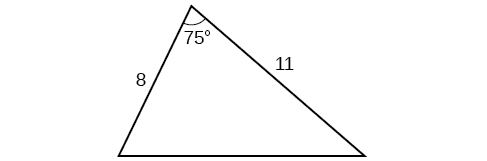A pilot is flying over a straight highway. He determines the angles of depression to two mileposts, 2.1 km apart, to be 25° and 49°, as shown in [link] . Find the distance of the plane from point $\text{\hspace{0.17em}}A\text{\hspace{0.17em}}$ and the elevation of the plane.

distance of the plane from point $\text{\hspace{0.17em}}A:\text{\hspace{0.17em}}$ 2.2 km, elevation of the plane: 1.6 km

## Non-right Triangles: Law of Cosines

Solve the triangle, rounding to the nearest tenth, assuming $\text{\hspace{0.17em}}\alpha \text{\hspace{0.17em}}$ is opposite side $\text{\hspace{0.17em}}a,\beta \text{\hspace{0.17em}}$ is opposite side $\text{\hspace{0.17em}}b,\text{\hspace{0.17em}}$ and $\text{\hspace{0.17em}}\gamma \text{\hspace{0.17em}}$ s opposite side

Solve the triangle in [link] , rounding to the nearest tenth.

$B=71.0°,C=55.0°,a=12.8$

Find the area of a triangle with sides of length 8.3, 6.6, and 9.1.

To find the distance between two cities, a satellite calculates the distances and angle shown in [link] (not to scale). Find the distance between the cities. Round answers to the nearest tenth.

40.6 km

## Polar Coordinates

Plot the point with polar coordinates $\text{\hspace{0.17em}}\left(3,\frac{\pi }{6}\right).$

Plot the point with polar coordinates $\text{\hspace{0.17em}}\left(5,-\frac{2\pi }{3}\right)$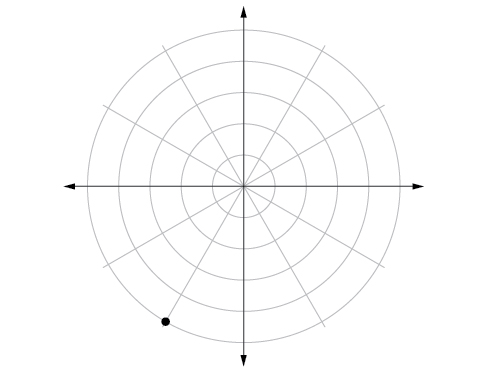Convert $\text{\hspace{0.17em}}\left(6,-\frac{3\pi }{4}\right)\text{\hspace{0.17em}}$ to rectangular coordinates.

Convert $\text{\hspace{0.17em}}\left(-2,\frac{3\pi }{2}\right)\text{\hspace{0.17em}}$ to rectangular coordinates.

$\text{\hspace{0.17em}}\left(0,2\right)\text{\hspace{0.17em}}$

Convert $\left(7,-2\right)$ to polar coordinates.

Convert $\left(-9,-4\right)$ to polar coordinates.

$\left(9.8489,203.96°\right)$

For the following exercises, convert the given Cartesian equation to a polar equation.

$x=-2$

${x}^{2}+{y}^{2}=64$

$r=8$

${x}^{2}+{y}^{2}=-2y$

For the following exercises, convert the given polar equation to a Cartesian equation.

$r=7\text{cos}\text{\hspace{0.17em}}\theta$

${x}^{2}+{y}^{2}=7x$

$r=\frac{-2}{4\mathrm{cos}\text{\hspace{0.17em}}\theta +\mathrm{sin}\text{\hspace{0.17em}}\theta }$

For the following exercises, convert to rectangular form and graph.

$\theta =\frac{3\pi }{4}$

$y=-x$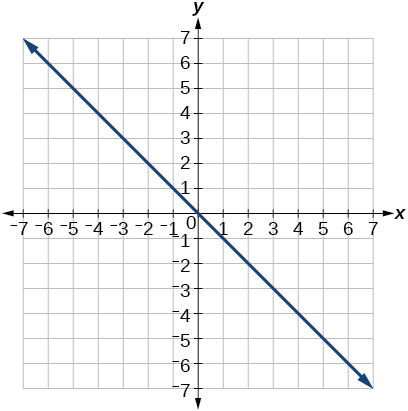$r=5\mathrm{sec}\text{\hspace{0.17em}}\theta$

## Polar Coordinates: Graphs

For the following exercises, test each equation for symmetry.

$r=4+4\mathrm{sin}\text{\hspace{0.17em}}\theta$

symmetric with respect to the line $\theta =\frac{\pi }{2}$

$r=7$

Sketch a graph of the polar equation $\text{\hspace{0.17em}}r=1-5\mathrm{sin}\text{\hspace{0.17em}}\theta .\text{\hspace{0.17em}}$ Label the axis intercepts.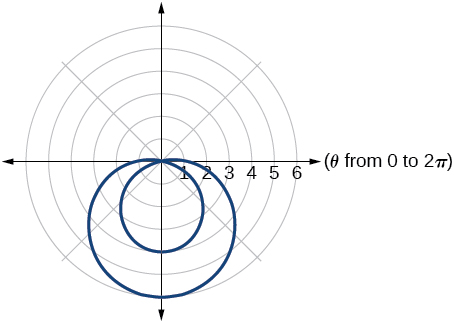Sketch a graph of the polar equation $\text{\hspace{0.17em}}r=5\mathrm{sin}\left(7\theta \right).$

Sketch a graph of the polar equation $\text{\hspace{0.17em}}r=3-3\mathrm{cos}\text{\hspace{0.17em}}\theta$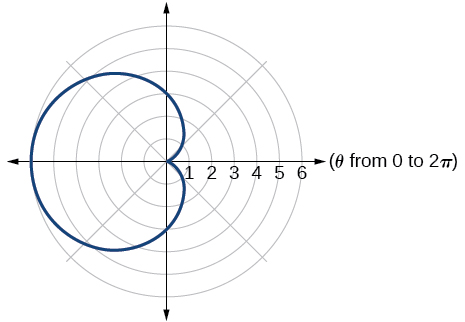## Polar Form of Complex Numbers

For the following exercises, find the absolute value of each complex number.

$-2+6i$

$4-\text{​}3i$

5

Write the complex number in polar form.

$5+9i$

$\frac{1}{2}-\frac{\sqrt{3}}{2}\text{​}i$

$\mathrm{cis}\left(-\frac{\pi }{3}\right)$

For the following exercises, convert the complex number from polar to rectangular form.

$z=5\mathrm{cis}\left(\frac{5\pi }{6}\right)$

$z=3\mathrm{cis}\left(40°\right)$

$2.3+1.9i$

For the following exercises, find the product $\text{\hspace{0.17em}}{z}_{1}{z}_{2}\text{\hspace{0.17em}}$ in polar form.

${z}_{1}=2\mathrm{cis}\left(89°\right)$

${z}_{2}=5\mathrm{cis}\left(23°\right)$

${z}_{1}=10\mathrm{cis}\left(\frac{\pi }{6}\right)$

${z}_{2}=6\mathrm{cis}\left(\frac{\pi }{3}\right)$

$60\mathrm{cis}\left(\frac{\pi }{2}\right)$

For the following exercises, find the quotient $\text{\hspace{0.17em}}\frac{{z}_{1}}{{z}_{2}}\text{\hspace{0.17em}}$ in polar form.

${z}_{1}=12\mathrm{cis}\left(55°\right)$

${z}_{2}=3\mathrm{cis}\left(18°\right)$

${z}_{1}=27\mathrm{cis}\left(\frac{5\pi }{3}\right)$

${z}_{2}=9\mathrm{cis}\left(\frac{\pi }{3}\right)$

$3\mathrm{cis}\left(\frac{4\pi }{3}\right)$

For the following exercises, find the powers of each complex number in polar form.

Find $\text{\hspace{0.17em}}{z}^{4}\text{\hspace{0.17em}}$ when $\text{\hspace{0.17em}}z=2\mathrm{cis}\left(70°\right)$

Find $\text{\hspace{0.17em}}{z}^{2}\text{\hspace{0.17em}}$ when $\text{\hspace{0.17em}}z=5\mathrm{cis}\left(\frac{3\pi }{4}\right)$

$25\mathrm{cis}\left(\frac{3\pi }{2}\right)$

difference between calculus and pre calculus?
give me an example of a problem so that I can practice answering
x³+y³+z³=42
Robert
dont forget the cube in each variable ;)
Robert
of she solves that, well ... then she has a lot of computational force under her command ....
Walter
what is a function?
I want to learn about the law of exponent
explain this
what is functions?
A mathematical relation such that every input has only one out.
Spiro
yes..it is a relationo of orders pairs of sets one or more input that leads to a exactly one output.
Mubita
Is a rule that assigns to each element X in a set A exactly one element, called F(x), in a set B.
RichieRich
If the plane intersects the cone (either above or below) horizontally, what figure will be created?
can you not take the square root of a negative number
No because a negative times a negative is a positive. No matter what you do you can never multiply the same number by itself and end with a negative
lurverkitten
Actually you can. you get what's called an Imaginary number denoted by i which is represented on the complex plane. The reply above would be correct if we were still confined to the "real" number line.
Liam
Suppose P= {-3,1,3} Q={-3,-2-1} and R= {-2,2,3}.what is the intersection
can I get some pretty basic questions
In what way does set notation relate to function notation
Ama
is precalculus needed to take caculus
It depends on what you already know. Just test yourself with some precalculus questions. If you find them easy, you're good to go.
Spiro
the solution doesn't seem right for this problem
what is the domain of f(x)=x-4/x^2-2x-15 then
x is different from -5&3
Seid
All real x except 5 and - 3
Spiro
***youtu.be/ESxOXfh2Poc
Loree
how to prroved cos⁴x-sin⁴x= cos²x-sin²x are equal
Don't think that you can.
Elliott
By using some imaginary no.
Tanmay
how do you provided cos⁴x-sin⁴x = cos²x-sin²x are equal
What are the question marks for?
ElliottByByBy Laurence BailenBy Mariah HauptmanBy OpenStaxBy Anonymous UserBy Brooke DelaneyBy Richley CrapoBy Stephen VoronBy Robert MurphyBy OpenStaxBy OpenStax